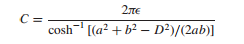### Create an Account

Home / Questions / Construct a curvilinear square map of the potential field between two parallel circular cy...

# Construct a curvilinear square map of the potential field between two parallel circular cylinders one of 4 cm radius inside another of 8 cm radius The two axes are displaced by 25 cm These

Construct a curvilinear-square map of the potential field between two parallel circular cylinders, one of 4 cm radius inside another of 8 cm radius. The two axes are displaced by 2.5 cm. These dimensions are suitable for the drawing. As a check on the accuracy, compute the capacitance per meter from the sketch and from the exact expression:Jun 14 2020 View more View LessSubscribe To Get Solution